# 声明

其实吧，这篇文章已经跟《三体》小说没有太大的关系了，这纯粹是一篇关于数学的文章，但是由于本文要涉及大量关于“趋势的趋势的趋势”，“走势的走势的走势的走势”，“导数的导数的导数的导数的导数…”，为了保持一致性，我将本文的题目写成了“《三体》读后感的读后感…”，可能后面还有，未完待续！

# 第一部分.泰勒展开解释

很多人都看过双截棍表演，但现如今很少有人了解鞭术了，其实你可以把鞭子看成是N趋近于无穷大时的N截棍，玩起来更难。其实我也不是很懂，就是为了解释这个泰勒展开才稍微看了一点关于鞭术的东西，具体来讲，执鞭人手执鞭子在原地只是上下左右按照一定的规则甩鞭，一条很长的鞭子就会整体展现成各种漂亮的曲线，他是怎么做到的？
当然，从物理上讲，这当然是若干列波从执鞭处向鞭子的另一端传播，传播的过程在不同的点产生了定向的效果，然而似乎不是一个很好的足以让人满意的解释，我们的问题是，那个执鞭人的手需要怎么个动作，才能让鞭子整体上看来是那种效果？
这个问题我是回答不了，因为我不懂鞭术，身边也没有懂的人，但是这个问题似乎和本文一开始的那个问题讲的是同一回事，即从一个点来蕴含整体的行为。

我的观点是：既然走势可以让人预测曲线上邻接的下一点的大致位置，那么走势的走势便可以相对精确地预测邻接下一点的具体位置，紧接着，走势的走势的走势便可以告诉人们这种趋势可以延续到什么时候，再继续…这似乎超出了人们的想象力…我们还是用简单的数学来表示吧。我们先从1阶导数，2阶导数，3阶导数的几何意义说起。
先看1阶导数，我们知道，它是经过曲线上某点的切线的斜率：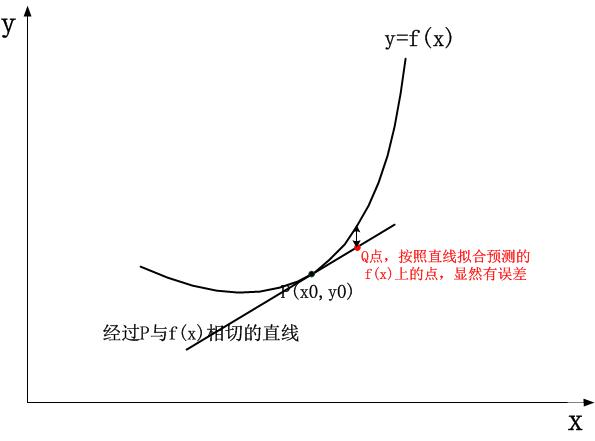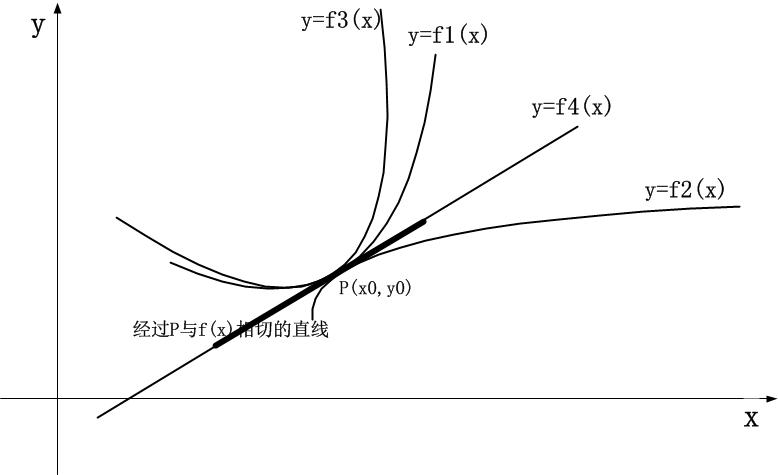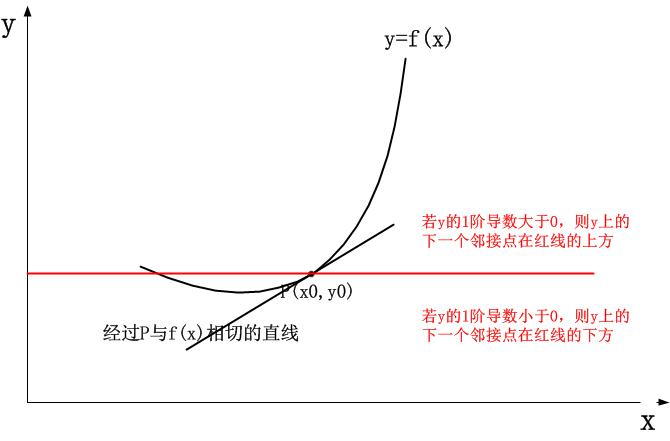so，我们需要进一步的信息，我们继续求2阶导数，看看能挖掘出什么新玩意儿。
2阶导数是1阶导数的导数，换句话说，它代表了检测点切线的变化趋势，有了这个趋势，我们是不是可以相对精确地预测邻接的点的位置了呢？我们先看2阶导数的几何意义为何。学过数学的都知道，2阶导数表示了曲线的凸凹，对于凸函数，2阶导数是负数，它表示切线的斜率会越来越小，而对于凹函数，2阶导数是正数，它表示切线的斜率越来越大：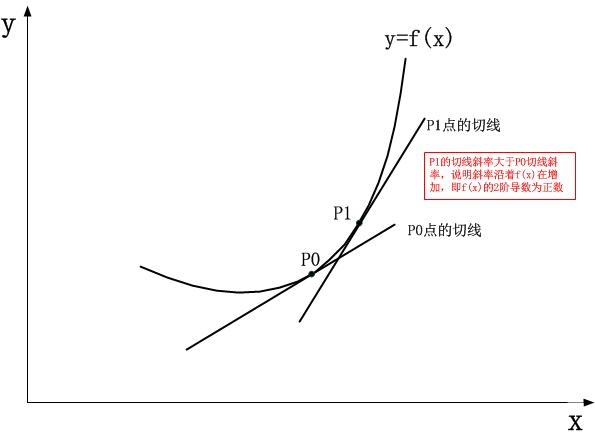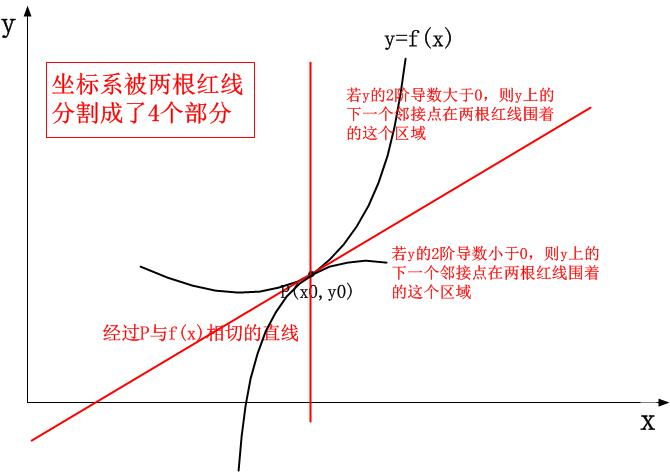到了这一步，进一步将曲线往前延伸似乎是无望的，因为：

1.首先，我们不知道代表检测点凸凹性的2阶导数的值在将来会不会逆转，即我们不知道曲线会不会由凸变凹或者由凹变凸；
2.其次，即便假设函数的凸凹性不变，我们也不知道接下来曲线是越来越凸/凹呢？还是反过来呢？

我们继续看3阶导数。在此之前，我必须要澄清一个我的观点。
我在知乎上查过相关资料，另外还特意请教过一些搞数学的老师或者朋友，得到的解答可能都是从哪个地方看到的一致性解答，说3阶，4阶，5阶…导数这些没有几何意义和物理意义，数学只追求逻辑上的完整，自包容，而不是去追求什么几何意义，物理意义。我并不赞成这个说法，以霍金为例，它的虚时间模型虽然只是数学上的技巧，但是最终的目标却是为他的有限无界的宇宙几何模型服务，这说明，完成逻辑完整性证明和寻找其意义同等重要，可能后者还会更重要，我没有看到哪一个伟大的物理学发现背后仅仅是纯粹的思辨性的数学，不管是牛顿的引力场，还是爱因斯坦的引力场，还是霍金的量子引力，在逻辑严谨性支撑的前脸，都有一个漂亮得体的几何模型作为表象。

3阶导数不难求，继续对2阶导数表达式求导，然后代入检测点的x值即可，然而3阶导数的意义是什么？其实仔细想想，并不难理解，这正如2阶导数主导1阶导数的变化从而把1阶导数自认为正确的“以直代曲”的直线模拟拉成弯曲的或者凸或者凹的曲线一样，3阶导数同样主导2阶导数的变化，它可以表示“曲线是继续凸下去或者继续凹下去，还是会在某一个x值后逆转，由凸变凹或者由凹变凸”。用语言表示比较苍白难以理解，于是我画个图示：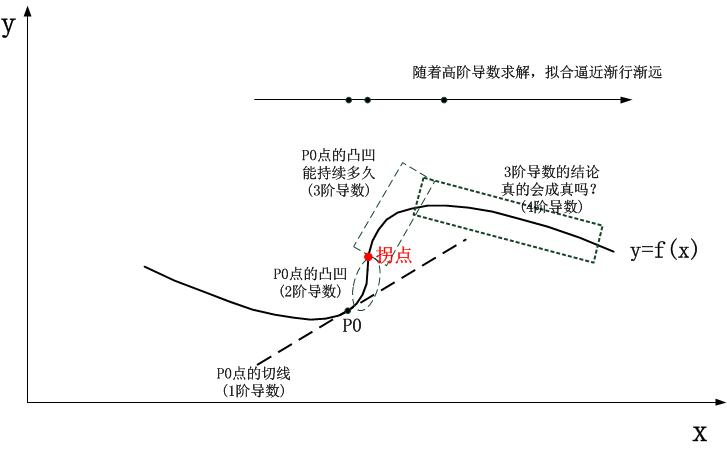不一定！But why？
虽然当前检测点的3阶导数值为-1，但这并不意味着它会一直保持-1，如果它一直保持-1，那么我们的预测正确，但是如果曲线的3阶导数在该检测点是递增的呢？这意味着会发生下面的情况：

上面的判断简直可以弥补3阶导数的误差，曲线进一步前进，很帅！那么如何判断3阶导数的走势呢？简单，求4阶导数！

我来画一个实际的例子结束讨论：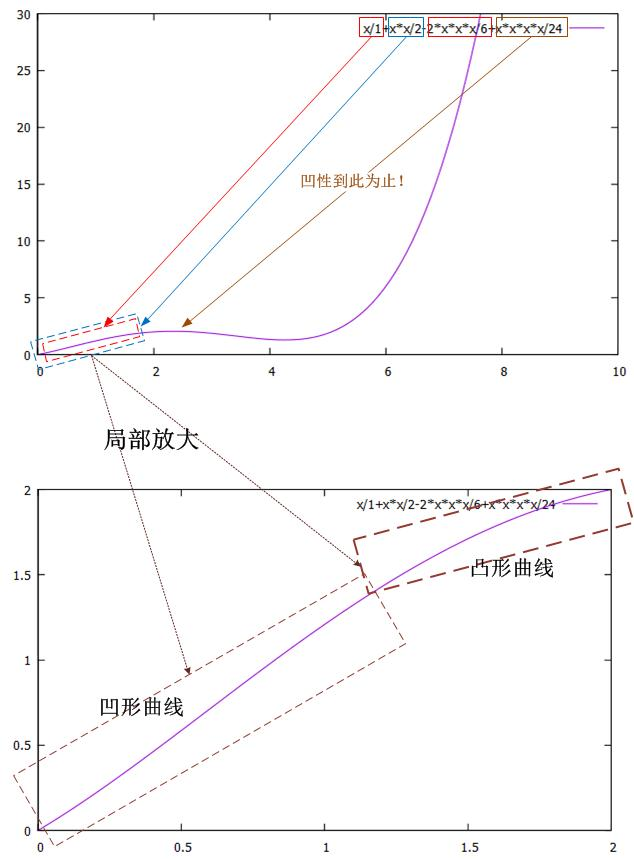在了解了曲线的走势后，剩下的就是用二项式去拟合了。其实，这种二项式叠加的拟合方式并没有什么特殊的含义，只是因为它是可以做到的而已，你同样可以用傅立叶变换的方式将一个函数在频域上展开，因为那也是一种可能的方式。其实任何两个或者多个带有实际效应的表达式叠加在一起，整体而言都会表现出各个叠加体局部的性质，这里重要的是一个纯数学上的技巧，即如何确定二项式的系数，可以肯定的是系数跟各阶导数是相关的，剩下的问题就是待定系数法求解了，这并不是需要赘述的内容。
综上，在这个待定系数的角度，二项式拟合任何曲线实属凑出来的，因为凑出来的二项式叠加表达式的各阶导数值恰好等于原函数的各阶导数值。
然而这并不能让不断寻找意义的人满意，如果非要在纯数学之外去寻找这样做的意义，那么我们可以从中值定理入手去理解。说好了不谈这些诸如中值定理的，但事实上，想彻底理解一个数学概念，这些概念是避不开的，问题是，我们如何更简单地(而不是更复杂地)去理解它们。
以拉格朗日中值定理为例，它的中值定理是这样的：

f(x)x0,x,[x0,x],ξ[x0,x]使:f(x)=f(x0)+f(ε)(xx0)$如果函数f(x)在（x_0,x）上可导,[x_0,x]上连续,则必有一ξ∈[x_0,x]使得: f(x)=f(x_0)+f^ \prime(\varepsilon)(x-x_0)$

f(x)x0,x,[x0,x],ξ[x0,x]使:f(x)=f(x0)+f(x0)(xx0)+f(x0)2!(xx0)2+f(ε)3!(xx0)3$如果函数f(x)在（x_0,x）上可导,[x_0,x]上连续,则必有一ξ∈[x_0,x]使得: f(x)=f(x_0)+f\prime(x_0)(x-x_0)+\frac{f\prime\prime(x_0)}{2!}(x-x_0)^2+\frac{f^ {\prime\prime\prime}(\varepsilon)}{3!}(x-x_0)^3$

我来给出上式子的一个几何解释，虽然我们想象不到2阶导数依照其几何意义如何画出来，但是我们可以把原始的函数本身升一个维度，然后用积分的思想去理解2阶导数的几何意义。
理解我在说什么了吗？我的意思简单点说，就是积分式的1阶导数(其实是导函数)就是被积函数，被积函数的1阶导数就是积分式的2阶导数，而我们知道积分式是有几何意义的，它表示面积，而被积函数则表示曲线，再进一步积分式的2阶导数则表示曲线上某点切线的斜率…这样，相当于我们将2阶导数看成了切线斜率，将1阶导数看成了曲线本身，而原始函数看成了曲线与x$x$轴围成的面积：

Farea=f(x)=x0g(x)dx$F_{area}=f(x)=\int_0^xg(x) {\rm d}x$
f=g(x)$f\prime=g(x)$
f=g=线$f\prime\prime=g\prime=切线斜率$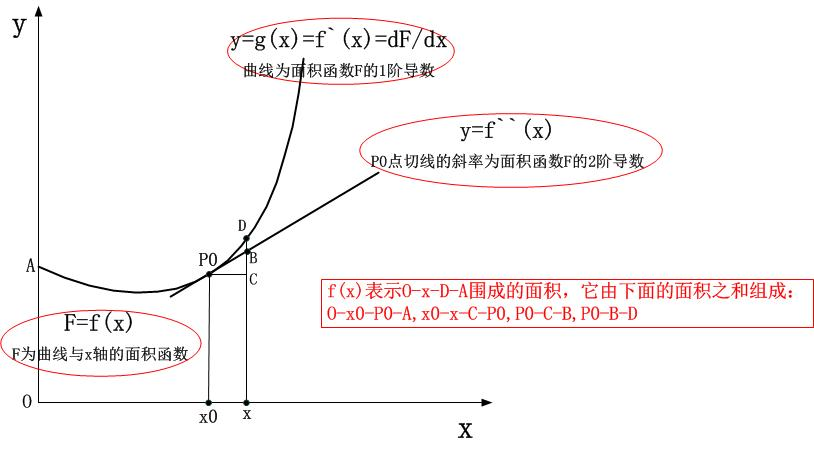SOxDA=SOx0P0A+Sx0xCP0+SP0CB+SP0BD$S_{OxDA}=S_{Ox_0P_0A}+S_{x_0xCP_0}+S_{P_0CB}+S_{P_0BD}$

SOxDA=f(x)=f(x0)+f(x0)(xx0)+SP0CB+SP0BD$S_{OxDA}=f(x)=f(x_0)+f\prime(x_0)(x-x_0)+S_{P_0CB}+S_{P_0BD}$

SOxDA=f(x)=f(x0)+f(x0)(xx0)+12f(x0)(xx0)+SP0BD$S_{OxDA}=f(x)=f(x_0)+f\prime(x_0)(x-x_0)+\frac{1}{2}f\prime\prime(x_0)(x-x_0)+S_{P_0BD}$

SOxDA=f(x)=f(x0)+f(x0)(xx0)+12f(x0)(xx0)+O(SP0BD)$S_{OxDA}=f(x)=f(x_0)+f\prime(x_0)(x-x_0)+\frac{1}{2}f\prime\prime(x_0)(x-x_0)+O(S_{P_0BD})$

好了，这就是一个在2阶情况下，中值定理大致的几何印象。循着这个思路推广下去，泰勒公式就在眼前了。
虽然我们想象不出来N$N$阶中值定理的几何意义，但是正像物理学中经常提及超维一样，我们也可以把二维的笛卡尔坐标系拓展成“无限维度空间中的超立方体”。这样的假设下，似乎还不是特别令人满意，但也就只能这样了。
事实上，我这里可以给出一点提示，利用积分中值定理可以证明泰勒公式在意义层面的合理性，利用分部积分从我上面给出的2阶导数直观几何意义开始，可以逐渐导出完整的泰勒公式！我不会在本文正文中去表达这些内容，因为怕公式太多。当你看线条找不出线条，夹角之间的关系时，试试面积，升个维度试试。
最后，记住一个结论，N$N$阶导数的几何意义物理意义要比如何用泰勒多项式表达任意表达式更加重要，后者只是说明它“恰好能做到”而已，除了泰勒多项式，傅立叶展开也可以达到同样的效果，另外，还可能有别的。
在观察二项式拟合任意函数的时候，我们知道“它恰恰可以做到”，现在的问题是如何感性的认识到这一点，即“它为什么就可以做到？”，我们以下面的两个多项式为例，来点感性认识：

f(x)=x2$f(x)=x^2$
f(x)=x3$f(x)=x^3$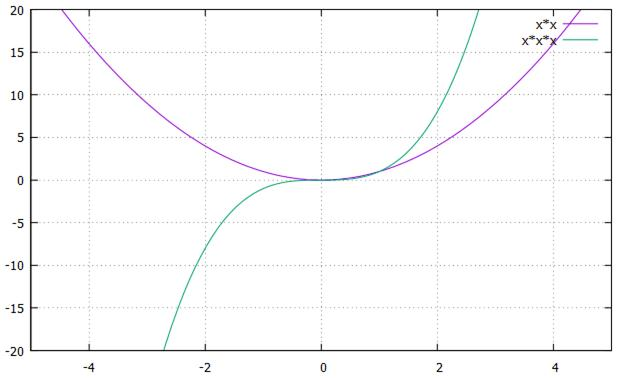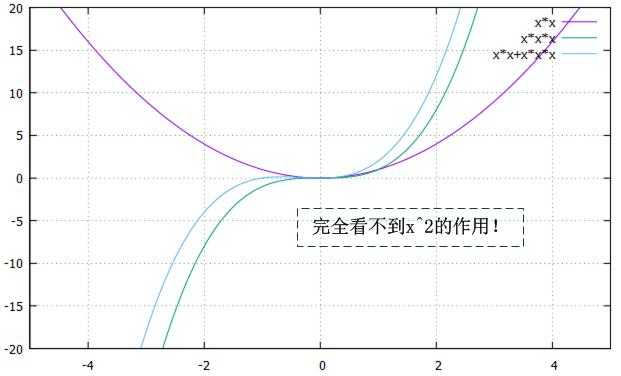然而，我们没有考虑缩放。
直观地看，虽然3次曲线在x$x$变化时，其y$y$值的变化剧烈程度要大于2次曲线(从其1阶导数上便能看得出，2次曲线是2x$2x$，3次曲线是3x2$3x^2$)，如果我们能适当地，恰到好处的把二者的差异通过缩放平滑掉，那么结果如何呢？考虑到我们只能用四则混合运算，非常简单，给3次多项式除以一个大于1的系数，减小它的y$y$效应，或者给2次多项式乘以一个大于1的系数，从而增加它的y$y$效应，我们来看看结果如何。先看缩小3次曲线效应的结果：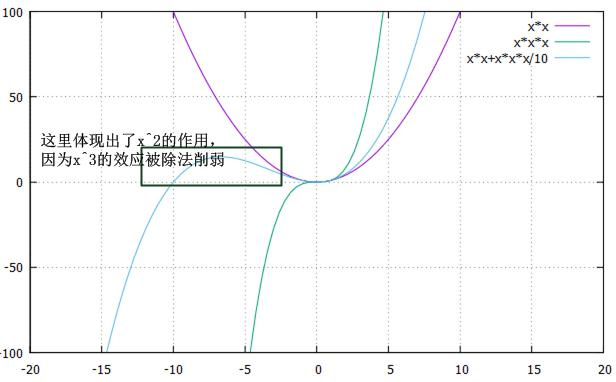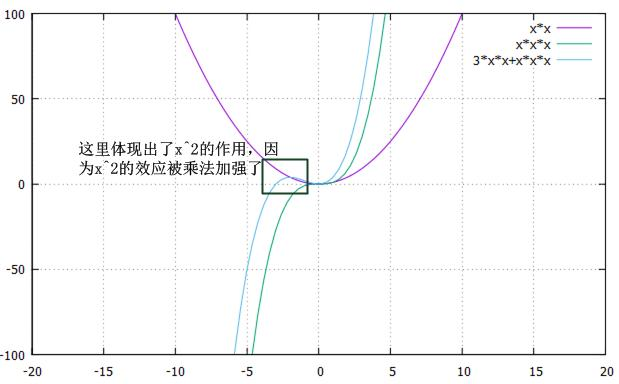到这里，我们知道，二项式的加和表达式是“可以”拟合任何曲线的，而泰勒展开式的那些系数则是一组“恰到好处”的系数，它保证了原表达式和展开表达式的各阶导数都是相等的！

我想，我终于把该表述的都说完了，有人提到说让我用动画去表示曲线的拟合，这个确实要比用图形和文字更加震撼，然而It is beyond my ability，我并不会这些东西，我所用的gnuplot都要折腾好久，我并没有什么更好的简单的工具来帮我做更加直观的东西，所以就只能通过画草图和文字加以赘述，实为能力所限，实在抱歉。
我们考虑一个形象的表述来结束关于这个话题的讨论，那就是牛顿定律的$\frac{位移}{时间}$公式：

v=at$v=at$
s=f(t)=v0t+12at2$s=f(t)=v_0t+\frac{1}{2}at^2$

st=vt0t$s_t = v_{t_0}t$

以上的讨论仅仅是在恒力作用下牛顿第二定律导出的结论，然而如果施加的力不是恒定的，而是一个关于时间t$t$变化的变力F=f(t)$F=f(t)$，那么此时仅仅2阶导数便不足以刻画时间t$t$后精确的位移了，此时要想预测时间t$t$后的位移，随着t$t$距离当前时间的远去，$\frac{位移}{时间}$的3阶导数，4阶导数…便派上了用场，注意，变化率的变化率使得我们能在越来越远的地方预测趋势，这个正是和上文中关于泰勒展开的讨论是一致的。

# 第二部分.另外一种刻画的方式

1.首先求解各阶导数，判断其增减性，凸凹性，极值，拐点等，并且描出这些点；
2.其次，拟合若干个可以判断的具体点。

不管怎样，虽然两种不同的画法在操作细节上是截然不一致的，但是结果是，两种方案的结论是完全一致的，函数被刻画了，性质坦然地舒展了，还有什么性质没有暴露呢？嗯，也许在无穷远处的无穷小的误差可能会引发逻辑上的争论。但此事并不经常，也不绝对。
在正文中我是通过一个单独的点来蕴含整体模式的，然而在数学上，多数的建议却是，采用了素描的方式从粗到细地去刻画。两类的效果是一致的。

# 第三部分.关于导数和频率

随着求导阶数的增加，效应频率也在不断降低，最终这些不同频率的效应将反馈到函数曲线上的任意一点，而这就完整勾勒出了整条曲线。如果能掌握了这个道理，那么那些执鞭者便可以从单点甩出任意曲线了，我们都知道，频率越高，传播距离越短，频率越低，传播距离越长，当然执鞭者也知道这个道理。这一切在数学上的反映，那就是：

以下是一个y=sin(x)$y=sin(x)$的泰勒展开的gif动画，红色代表原始的y=sin(x)$y=sin(x)$，绿色曲线的每一帧表示多了一阶的导数，也就是展开式中多了一项，可以看得出，随着求导的深入，绿色曲线将越来越拟合原始的正弦曲线，能拟合到什么程度呢？答案是“你想到什么程度，就能到什么程度”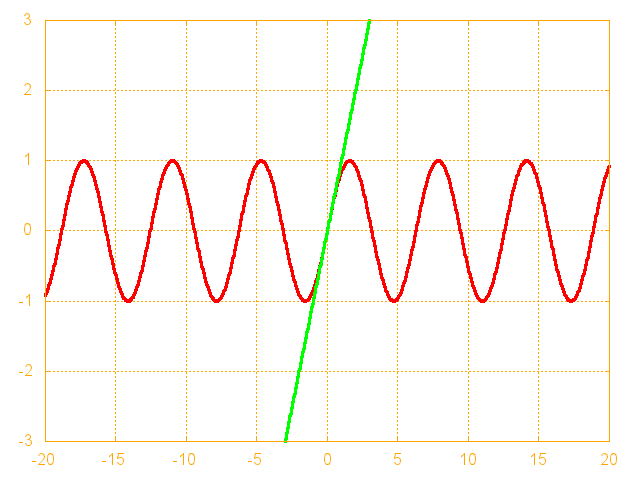# 附录

## A. 分部积分法推导泰勒公式

f(x)=f(x0)+f(x0)(xx0)+$f(x)=f(x_0)+f\prime(x_0)(x-x_0)+误差余项$

我们首先希望推倒多米诺骨牌的第一块试试看，升个维度，把导数写成积分的形式，我们有：

f(x)=f(x0)+xx0f(xr)dr$f(x)=f(x_0)+\int_{x_0}^xf\prime(x-r){\rm d}r$

udv=uvvdu$\int u{\rm d}v=uv-\int v{\rm d}u$
$现在设：$
u=f(xr)$u=f\prime(x-r)$
v=r$v=r$
$于是：$
xx0f(xr)dr=(f(xr)r)xx0xx0rdf(xr)$\int_{x_0}^x f\prime(x-r){\rm d}r=(f\prime(x-r)r)_{x_0}^x-\int_{x_0}^x r{\rm d}f\prime(x-r)$
$按照积分区间整理上式子：$
xx0f(xr)dr=f(x0)(xx0)+xx0f(xr)2dr2$\int_{x_0}^x f\prime(x-r){\rm d}r=f\prime(x_0)(x-x_0)+\int_{x_0}^x \frac{f\prime\prime(x-r)}{2}{\rm d}r^2$
$代入最终表达式：$
f(x)=f(x0)+f(x0)(xx0)+xx0f(xr)2dr2$f(x)=f(x_0)+f\prime(x_0)(x-x_0)+\int_{x_0}^x \frac{f\prime\prime(x-r)}{2}{\rm d}r^2$

f(xr)2dr2$\int \frac{f\prime\prime(x-r)}{2}{\rm d}r^2$

大致意思就是这样，过程就不详细写了。

## B.泰勒公式失效的场景(这个附录会很长)

是时候把物理意义扯出来了。
在正文中，我强调的那些可以“从一点窥见整个世界”的曲线其实有有条件的，那就是它的定义域必须是全体实数，且处处可导，若不是这样的话，情况就会复杂得多，这些复杂的情况在本附录讨论。在详细讨论前，有个声明。
由于本文并不是在同一时间写的，中间间隔了一个工作周，在这一周中我学会并喜欢上了Geogebra，所以我放弃了gnuplot，以下的图示全部来自于Geobebra。让我们开始吧！
首先看一个简单的反比例函数y=1x$y=\frac{1}{x}$，我们看下它的图像：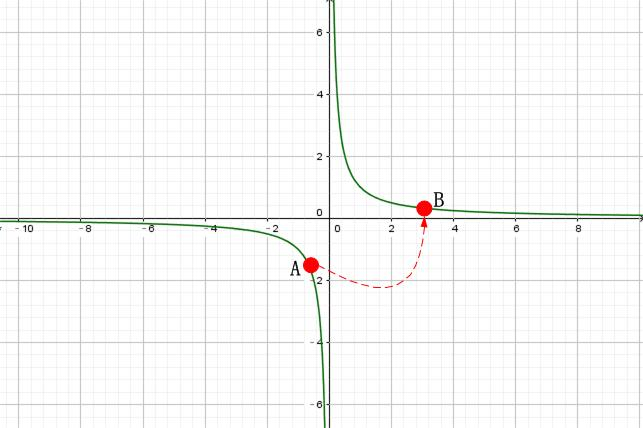奇点的意义在于，在该点，曲线是没有定义的，在这种点处，求导什么的都是毫无意义的，这些点真的就是“奇异”的点，任何计算，任何公式，定律都毫无意义(下文中将会赋予奇点以意义！)。
知道了奇点的概念后，再说一个例子。
有一天我微信上问温州皮鞋厂老板有没有什么好玩的东西，老板说算5$\sqrt{5}$比较好玩，然后我便犯了一个低级的错误。我信口开河“把y=x12$y=x^{\frac{1}{2}}$泰勒展开，把5$5$代入不就可以了吗”，为了在计算中不引入根号，瞬间想到了在x=1$x=1$处展开…计算是简便了，然而结果对吗？
我们先来看一段动画，即在x=1$x=1$处展开y=x12$y=x^{\frac{1}{2}}$的最多n=50$n=50$阶逼近的过程(Geogebra做这个非常帅！简单直接易上手！)：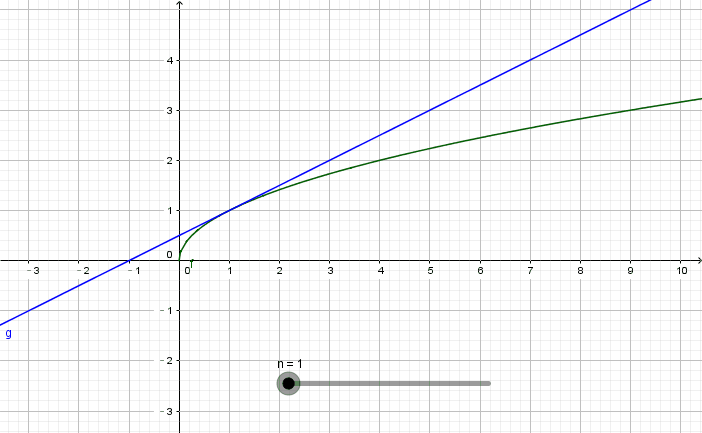n=1$n=1$n=50$n=50$，把x=5$x=5$代入均得不到正确的结果。可见，结论并不是我们之前预期的那样，在“x=1$x=1$这一个点窥见整条曲线”，显然“能窥见”的曲线范围仅仅局限在大致(0,2)$(0,2)$这个区间里。到底发生了什么？在进一步阐释物理意义之前，我还有点建议，如果你没有接触过复分析，收敛圆，收敛半径，审敛法这些，那么正好，如果你接触过这些但只是懂概念，能推导，那么建议暂时忘掉，如果你精通这些，那么不建议继续阅读下去。
………….

宇宙的膨胀意味着时间的膨胀和空间的膨胀，既然是膨胀，肯定需要多余的物质和能量，当我们吹气球的时候，气球之所以会膨胀，那是因为有气体不断地注入气球内部，同时气球球壁还有足够的厚度可以展开，总之，整个过程是需要物质(球壁)和能量(吹气)的！宇宙外面是什么并不是我们讨论的范围，霍金认为宇宙就是宇宙，是一个自洽的整体，那么显然没有在宇宙外面吹气的那个人，宇宙也不会有球壁…促使宇宙膨胀的物质和能量来自哪里？
来自奇点！
来自奇点！
来自奇点！
奇点早就蕴含了一切！
用霍金宇宙的奇点思想理解数学上的奇点概念，是朴素的。一条连续且光滑的曲线，它由它的奇点蕴含并生成，以y=1x$y=\frac{1}{x}$为例，它由两条连续且光滑的曲线构成，x=0$x=0$是该函数的一个奇点，也是唯一的奇点，那么x=0$x=0$处便蕴含并可生成整个两条曲线了。
你可以把奇点想象成一个无线维度浓缩在一起的一个点，就像面团一样，可以展开到任何维度，可以擀成饺子皮，可以拉成拉面，可以团成馒头…现在我们用奇点的概念来描述一下函数y=x12$y=x^{\frac{1}{2}}$的曲线的生成过程：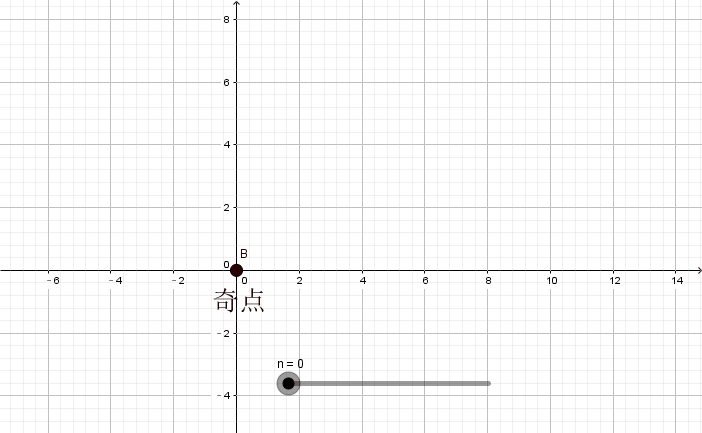由上面的过程性描述可知，如果将f(x)$f(x)$x=a$x=a$处泰勒展开了，那么泰勒公式仅仅能看到的是曲线从其奇点开始到x=a$x=a$结束这个区间的部分，其余的部分它是看不到的。这意味着什么？
这意味着，泰勒公式仅仅可以利用曲线来自奇点的从奇点到这个展开点之间的小区间的“物质”和“能量”，这些能量有多少决定了泰勒公式能量曲线往后(离开奇点的方向上)延展多远，如果说从奇点到泰勒展开点，能量已经释放了E$E$，那么泰勒公式就只能利用大小等于E$E$的能量去展开原函数：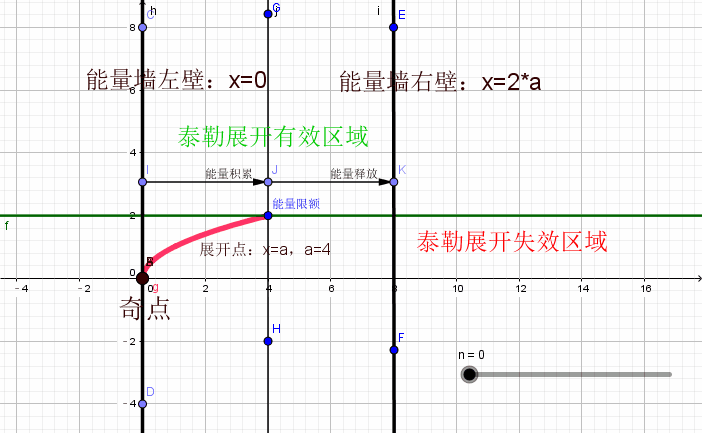当一个人讲话的时候，一些喜欢接话头的人就会插嘴说，我知道你想说什么，我来说吧。然而这种出风头的策略并非总是奏效。如果原来讲话的人刚开始说话，那么插话的人往往也预测不了太多的内容，然而如果最开始讲话的人说了很久，那么一些暗中观察的插话者往往能预测很多的内容并接着说下去。回到我们的泰勒展开，也就是说，展开点离开奇点越远，那么泰勒公式拟合的就越远，这背后的思想就是能量守恒，奇点已经将能量积累到了展开点，那么泰勒公式也就仅仅能用这么多能量(事实上所谓的能量就是各阶导数信息)来展开，这么多能量能跑多远呢？积累过程跑了多远，那就还能跑那么远！想想单摆运动吧，就这个道理。
那么y=sin(x)$y=sin(x)$y=ex$y=e^x$这些函数的奇点在哪里？这些函数的奇点在无穷远处。这意味着，在任意一个展开点，函数曲线本身都已经积累的无穷的能量(即信息)，这说明泰勒公式可以将其完全展开到无穷。
离奇点越近，各种趋势越不易表现出来，奇点附近刚开始积累趋势，趋势尚未表现，这就是能量和运动的观点，我就是这样避开了那些收敛圆，收敛半径这些概念的。

## C.到底什么是数学

(TODO)

©️2019 CSDN 皮肤主题: 编程工作室 设计师: CSDN官方博客